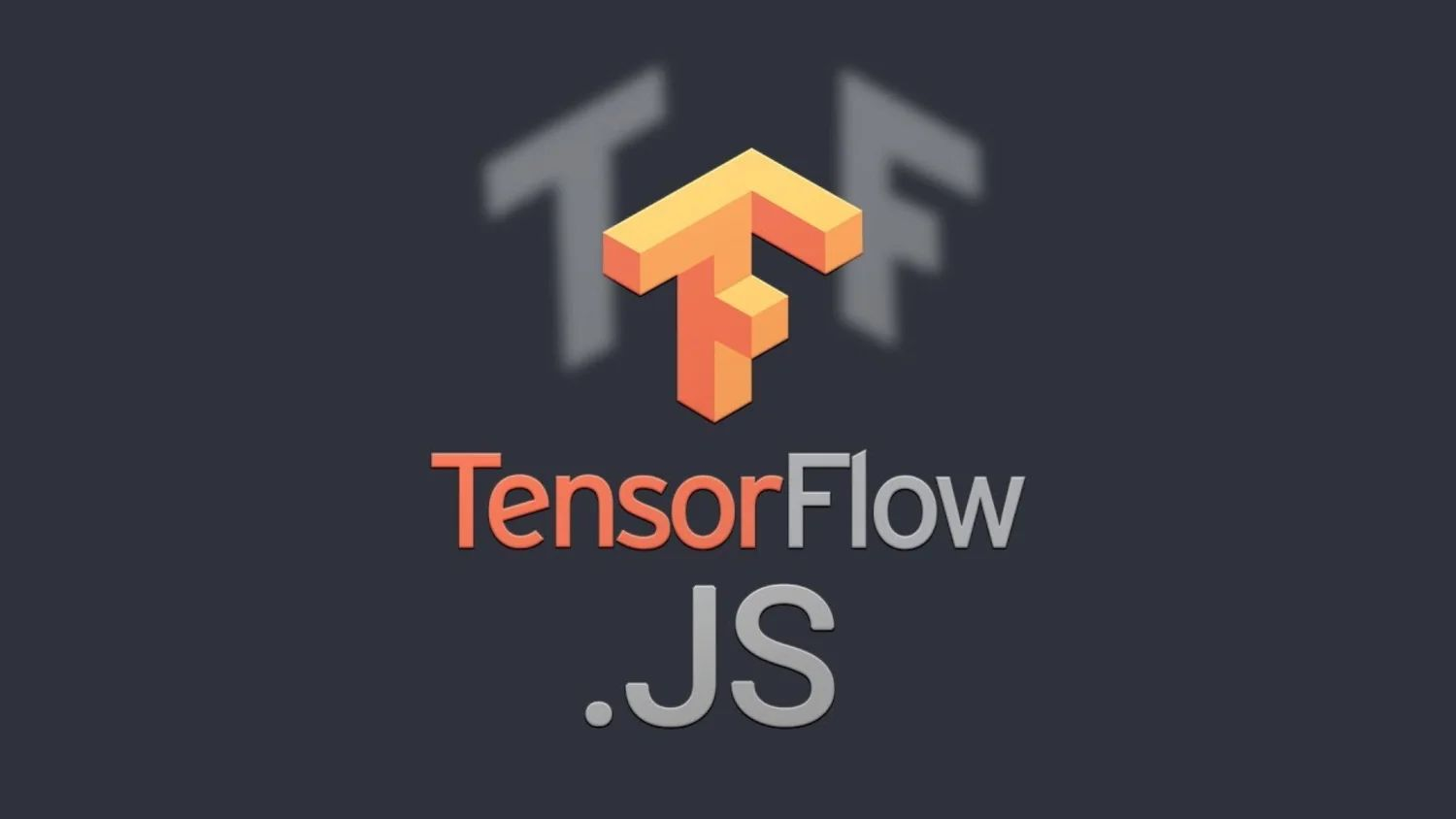# TensorFlow JS

##### Neural network implementation on a web browserAs a Javascript lover, and doing data science as my full time job, TensorFlow JS is like a dream come through, now you can run lightweight ML models directly in the web browser and share them!

# Why TensorFlow JS

• there is no installation, users can access your model via web browser, you can share your machine learning capability by hosting it on the web
• allowing users to train a model with their data without first handling to you

# Let’s begin

Open your favourite editor, create a new .html file (for example tfjs.html), and add the following code to your HTML file:

``````<html>
<script src="https://cdn.jsdelivr.net/npm/@tensorflow/tfjs@0.13.0"> </script>

<!-- Place your code in the script tag below. You can also use an external .js file -->
<script>
console.log('hi, this works');
</script>

<body>
</body>
</html>
``````

Here we are importing version 0.13, you can get the latest version at TensorFlow JS. Save the HTML file, and open the file in a web browser (i’m using Google Chrome). Press f12 for the developer’s console and click on Console tab. You should see hi, this works, if you don’t, refresh the page. In the Console, this is our interactive session just like Jupyter Notebook.

Lets declare a new model, a feed forward back propagation neural network call sequential.

``````const model = tf.sequential();
``````

You will see undefined and its fine, you can use the variable model.

Lets add (feed forward) fully connected layers to our model:

``````model.add(tf.layers.dense({units: 8, inputShape: }));
``````

Layers are building blocks for ML Model. Each layer performs a certain computation to transform its input to its output.

Next, lets specify the loss and the optimiser for the model to get some learning:

``````model.compile({loss: 'meanSquaredError', optimizer: 'sgd'});
``````

Now we need some training data:

``````const xs = tf.tensor2d([1, 2, 3, 4, 5], [5, 1]);
const ys = tf.tensor2d([1, 4, 9, 16, 25], [5, 1]);
``````

Tensor is the n-dimensional array that holds our data.

And time to fit our model:

``````model.fit(xs, ys, {epochs: 100, shuffle:true})
``````

This time you will get a promise. as training takes time, promise allows you to have an inner function to run codes after the current function is completed. you can read more about promise.

After the model has finish training, predict some values with:

``````model.predict(tf.tensor2d(, [1, 1])).print();
console.log(model.predict(tf.tensor2d(, [1, 1])).dataSync());
``````

.predict will return a Tensor, print() is a TFJS function that prints the value in a Tensor, and dataSync() returns the Float32Array.

So, this is the ‘hello world’ of TFJS, check out TFJS documentation.

# full code

``````<html>
<script src="https://cdn.jsdelivr.net/npm/@tensorflow/tfjs@0.12.0"> </script>
<script>
// Define a model for linear regression. model fit and predict
const model = tf.sequential();

// Prepare the model for training: Specify the loss and the optimizer.
model.compile({loss: 'meanSquaredError', optimizer: 'sgd'});

// Generate some synthetic data for training.
const xs = tf.tensor2d([1, 2, 3, 4, 5], [5, 1]);
const ys = tf.tensor2d([1, 4, 9, 16, 25], [5, 1]);

// Train the model using the data.
model.fit(xs, ys, {epochs: 1000, shuffle:true}).then(() => {
// Open the browser devtools to see the output
model.predict(tf.tensor2d(, [1, 1])).print();
model.predict(tf.tensor2d(, [1, 1])).print();
model.predict(tf.tensor2d(, [1, 1])).print();
model.predict(tf.tensor2d(, [1, 1])).print();
model.predict(tf.tensor2d(, [1, 1])).print();
model.predict(tf.tensor2d(, [1, 1])).print();
model.predict(tf.tensor2d(, [1, 1])).print();
});
</script>